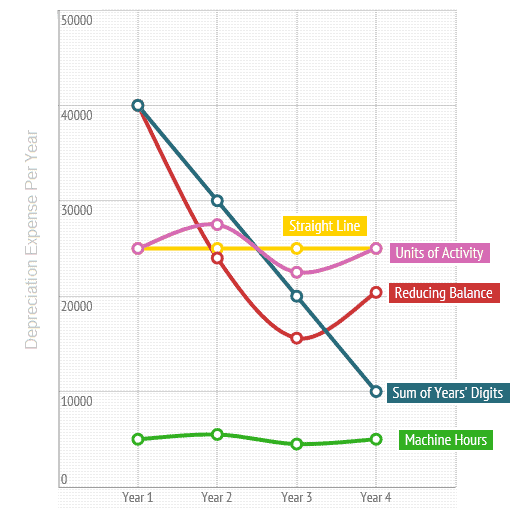# Depreciation Methods

Cost of a fixed asset must be charged to the income statement in a manner that best reflects the pattern of economic use of assets.

## Types of depreciation

Common methods of depreciation are as follows:

Same depreciation is charged over the entire useful life.

Depreciation expense decreases at a constant rate as the life of an asset progresses.

Depreciation charge declines by a constant amount as the life of the asset progresses.

Depreciation charge varies each period in proportion to the change in level of activity.

## Impact of using different depreciation methods

The total amount of depreciation charged over an asset’s entire useful life (i.e. depreciable amount) is the same irrespective of the choice of depreciation method. The adoption of a particular depreciation method does however effect the amount of depreciation expense charged in each year of an asset’s life.

Following diagram illustrates the effect of using different depreciation methods on yearly depreciation expense:The above illustration is based on the following information:

Cost of fixed asset

\$100,000

Residual Value

Nil

Useful Life

4 Years

Total Machine hours

20,000
(for calculating depreciation using units of activity method)

Rate of depreciation

40%
(for calculating depreciation using reducing balance method)

For calculation and working, you may view the depreciation worksheet.

Following can be deduced from the diagram:

Straight Line Depreciation

Results in an equal expense of \$25,000 each year.

Reducing Balance Depreciation

Depreciation charge is reduced by 40% in each period (i.e. the rate used in this example) until the last year in which the entire un-depreciated amount is charged off.

Sum of the Year' Digits Depreciation

Depreciation expense decreases each year by \$10,000.

Units of Activity Depreciation

Depreciation charge varies in line with the change in number of machine hours consumed each year.

## Comparison of Depreciation Methods

Easy to calculate
Useful where the pattern of economic benefits are hard to determine with precision.
Suitable for depreciating assets that provide similar level of economic benefits throughout their useful life (e.g. buildings).

May not reflect the true pattern of asset's economic benefits.

Appropriate where the usefulness of an asset declines over its useful life (e.g. IT equipment).

The rate of depreciation selected is subject to bias

Easier to understand.
The effect of decrease in depreciation expense compared to reducing balance method.

More difficult to calculate.

Most accurately reflects the pattern of consumption of economic benefits.
Suitable in case of fixed assets that depreciate in proportion to units of activity rather than just the passage of time.

Difficult to determine and measure a reasonable basis of activity.

View detailed explanation, examples and exercises of each depreciation method by selecting related topics below.